## How to Calculate and Solve for Rated Static Head (for Gating at Mould Parting) | Design of Gating System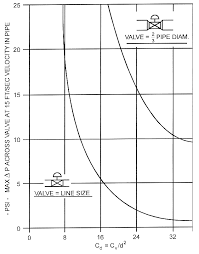The image above represents rated static head (for Gating at Mould Parting).

To compute for rated static head, two essential parameters are needed and these parameters are Height of the Sprue from its Top to the Point of Metal Entry into the Mould Cavity (H) and Height of the Casting (C).

The formula for calculating rated static head (for gating at mould parting).

Hs = H – C8

Where:

Hs = Rated Static Head (for gating at mould parting)
H = Height of the Sprue from its Top to the Point of Metal Entry into the Mould Cavity
C = Height of Casting

Let’s solve an example;
Find the rated static head (for gating at mould parting) when the height of the sprue from its top to the point of metal entry into the mould cavity is 11 and the height of casting is 19.

This implies that;

H = Height of the Sprue from its Top to the Point of Metal Entry into the Mould Cavity = 11
C = Height of Casting = 19

Hs = H – C8
Hs = 11 – 19 / 8
Hs = 11 – 2.375
Hs = 8.625

Therefore, the rated static head (for gating at mould parting) is 8.625 m.

Calculating the Height of the Sprue from its Top to the Point of Metal Entry into the Mould Cavity when the Rated Static Head (for gating at mould parting) and the Height of Casting is Given.

H = Hs + C8

Where;

H = Height of the Sprue from its Top to the Point of Metal Entry into the Mould Cavity
Hs = Rated Static Head (for gating at mould parting)
C = Height of Casting

Let’s solve an example;
Find the height of the sprue from its top to the point of metal entry into the mould cavity when the rated static head is 22 and the height of casting is 8.

This implies that;

Hs = Rated Static Head (for gating at mould parting) = 22
C = Height of Casting = 8

H = Hs + C8
H = 22 + 88
H = 22 + 1
H = 23

Therefore, the height of the sprue from its top to the point of metal entry into the mould cavity is 23 m.

Calculating the Height of Casting when the Rated Static Head (for gating at mould parting) and the Height of the Sprue from its Top to the Point of Metal Entry into the Mould Cavity is Given.

c = – (Hs – H) 8

Where;

C = Height of Casting
Hs = Rated Static Head (for gating at mould parting)
H = Height of the Sprue from its Top to the Point of Metal Entry into the Mould Cavity

Let’s solve an example;
Find the height of casting when the rated static head is 40 and the height of the sprue from its top of the point of metal entry into the mould cavity is 24.

This implies that;

Hs = Rated Static Head (for gating at mould parting) = 40
H = Height of the Sprue from its Top to the Point of Metal Entry into the Mould Cavity = 24

c = – (Hs – H) 8
c = – (40 – 24) 8
c = – (16) 8
c = – 128

Therefore, the height of casting is – 128 m.

Nickzom Calculator – The Calculator Encyclopedia is capable of calculating the rated static head (for gating at mould parting).

To get the answer and workings of the rated static head (for gating at mould parting) using the Nickzom Calculator – The Calculator Encyclopedia. First, you need to obtain the app.

You can get this app via any of these means:

To get access to the professional version via web, you need to register and subscribe for NGN 1,500 per annum to have utter access to all functionalities.
You can also try the demo version via https://www.nickzom.org/calculator

Apple (Paid) – https://itunes.apple.com/us/app/nickzom-calculator/id1331162702?mt=8
Once, you have obtained the calculator encyclopedia app, proceed to the Calculator Map, then click on Materials & Metallurgical under Engineering.Now, Click on Foundry Technology under Material & MetallurgicalNow, Click on Design of Gating System under Foundry Technology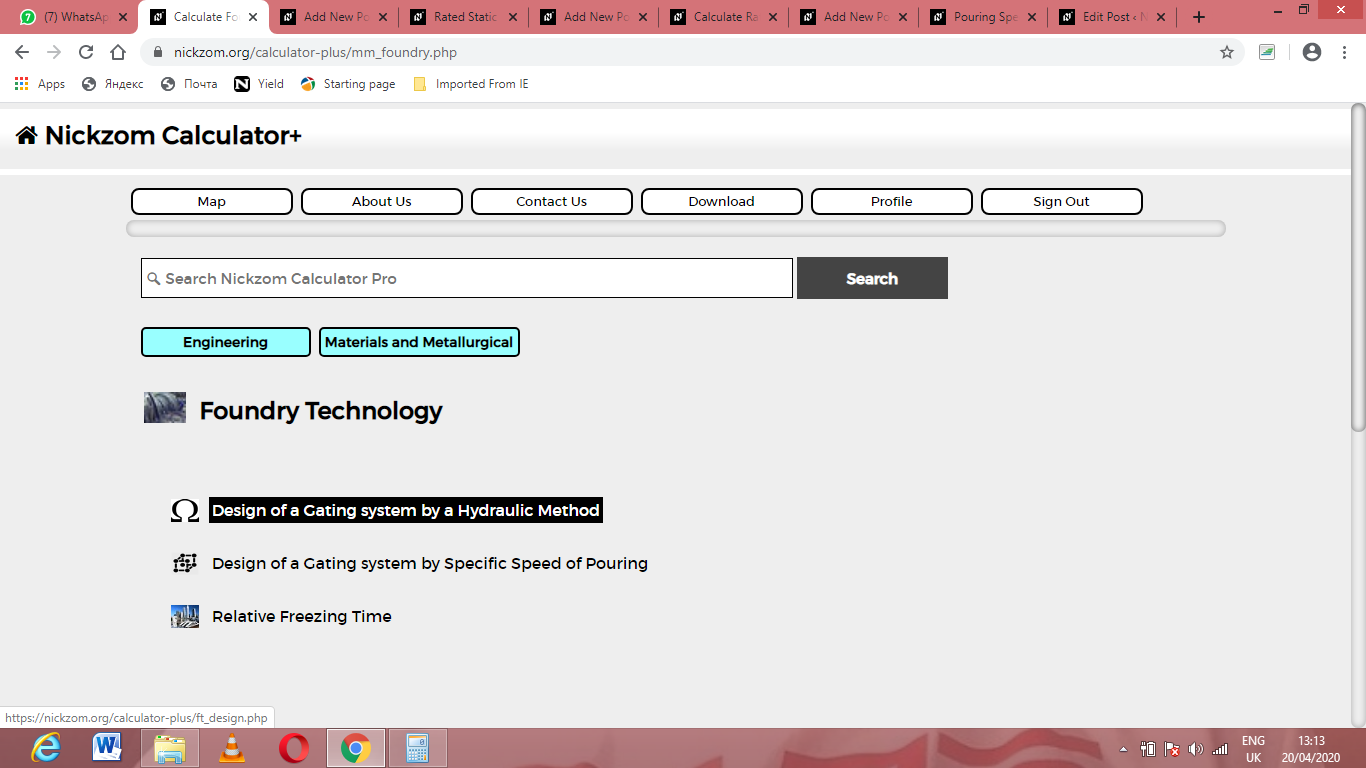Now, Click on Rated Static Head (for gating at mould parting) under Design of Gating System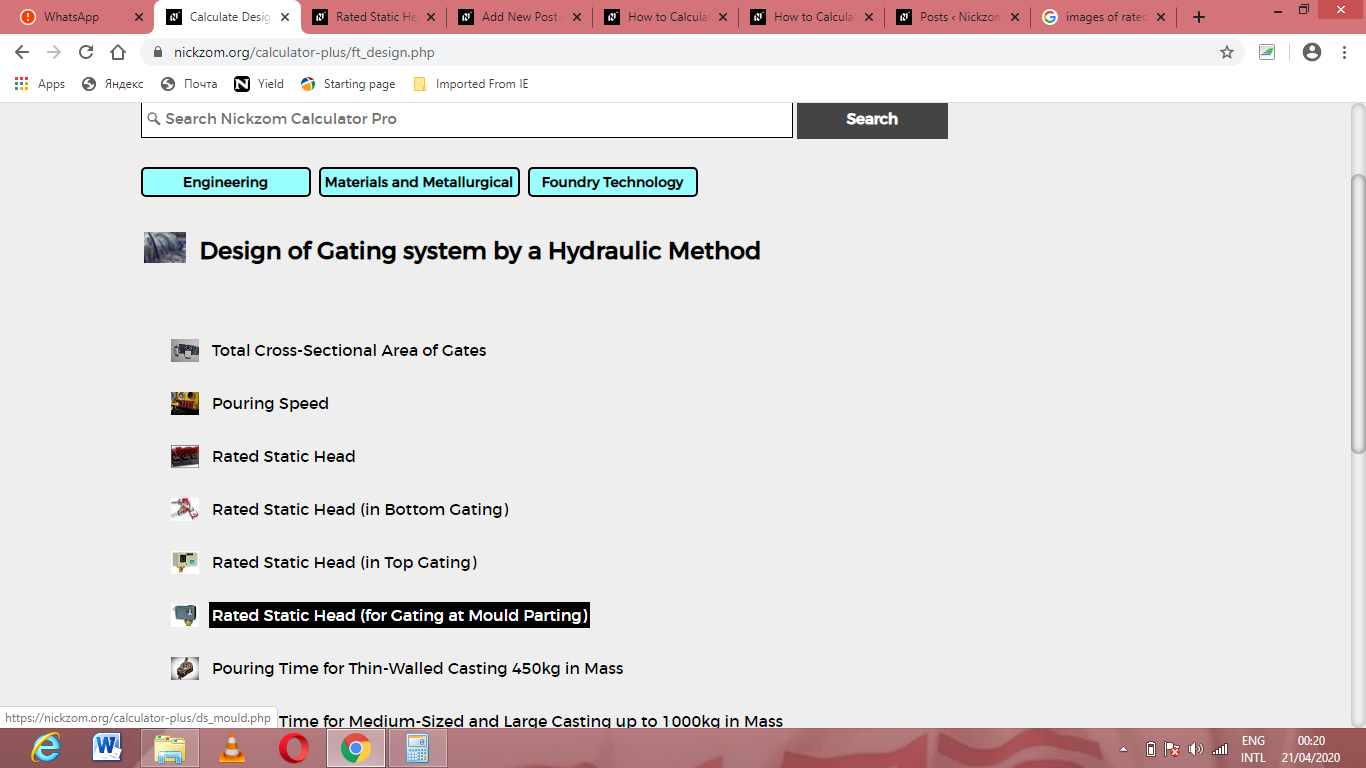The screenshot below displays the page or activity to enter your values, to get the answer for the rated static head (for gating at mould parting) according to the respective parameters which are the Height of the Sprue from its Top to the Point of Metal Entry into the Mould Cavity (H) and Height of the Casting (C).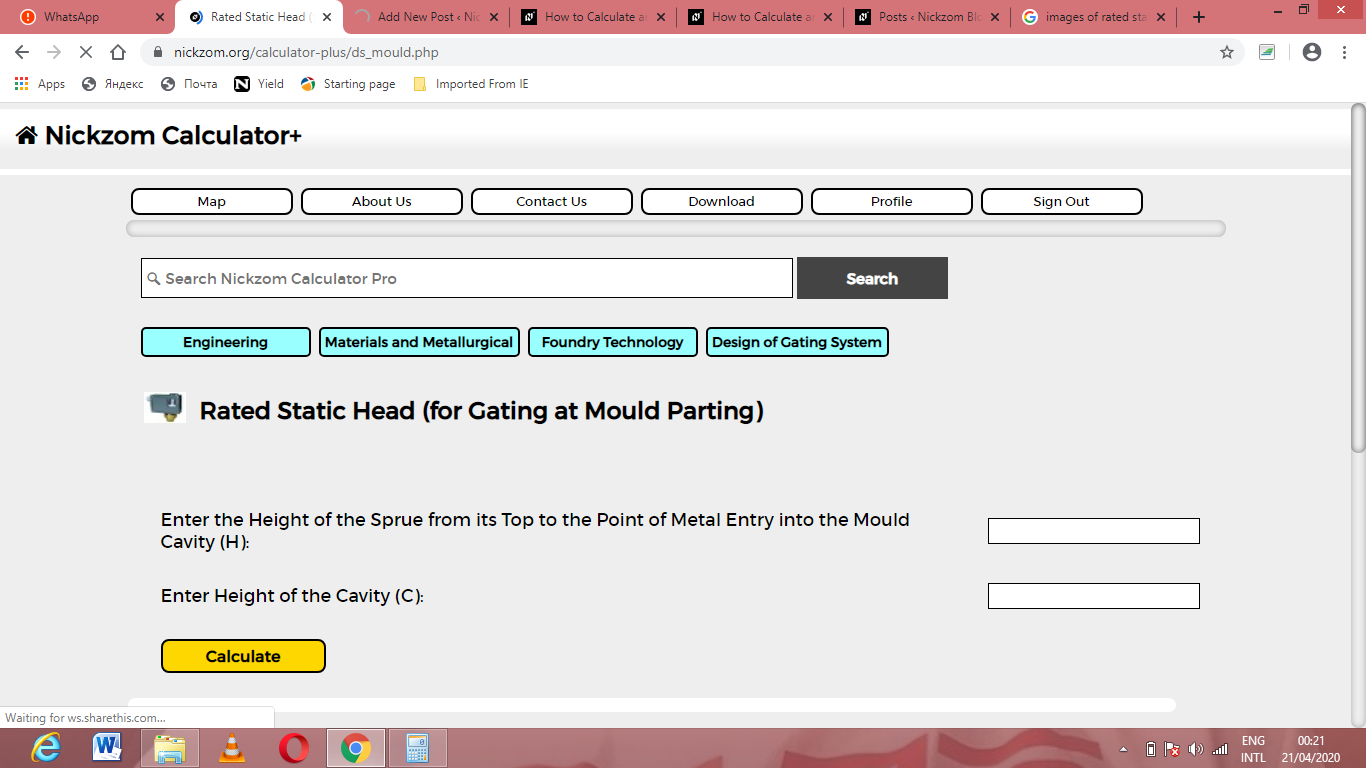Now, enter the values appropriately and accordingly for the parameters as required by the Height of the Sprue from its Top to the Point of Metal Entry into the Mould Cavity (H) is 11 and Height of the Casting (C) is 19.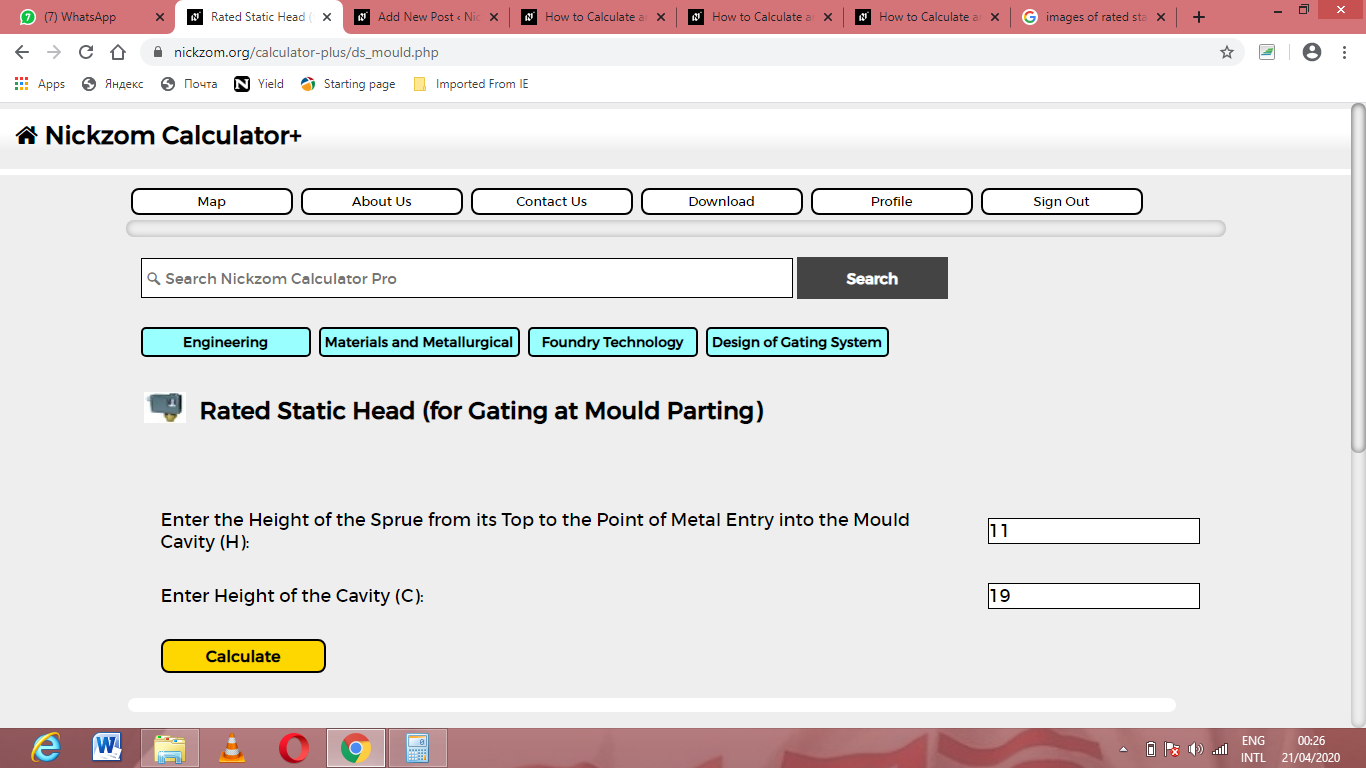Finally, Click on Calculate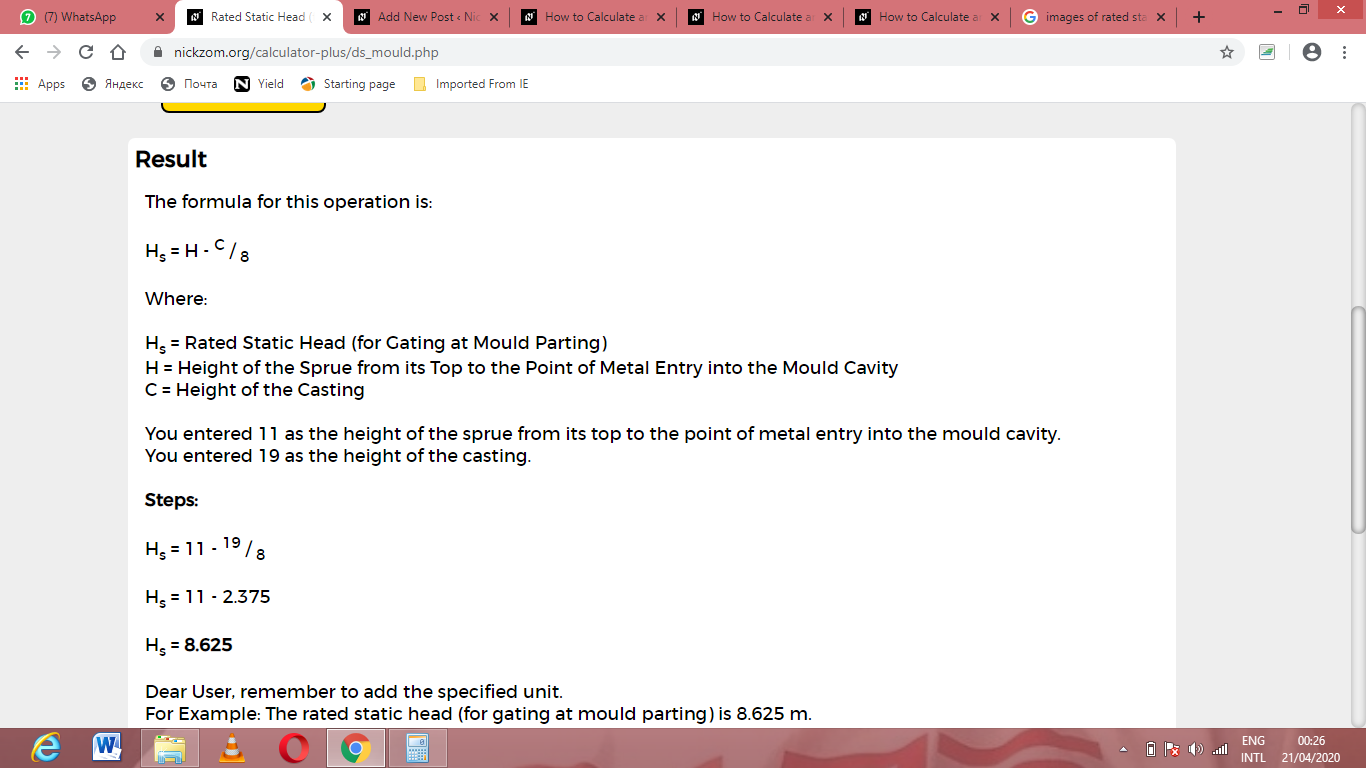As you can see from the screenshot above, Nickzom Calculator– The Calculator Encyclopedia solves for the rated static head (for gating at mould parting) and presents the formula, workings and steps too.